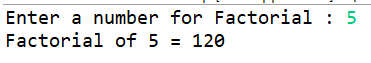## Different ways to Find Factorial of a Number

Category : Java | Sub Category : Java Programs | By Runner Dev Last updated: 2020-10-03 20:30:57 Viewed : 242

# Different ways to Find Factorial of a Number

## 2.  using while loop

FactorialwhileLoop .java

package runnerdev;

import java.util.Scanner;

public class FactorialwhileLoop {

public static void main(String[] args) {

System.out.print("Enter a number for Factorial : ");

Scanner sc = new Scanner(System.in);

int givenNumber = sc.nextInt();

int i = 1;

long factorial = 1;

while (i <= givenNumber) {

factorial *= i;

i++;

}

System.out.printf("Factorial of %d = %d", givenNumber, factorial);

}

}

Out put:## 3.  using Java8 API

##### java.util.stream.LongStream

A sequence of primitive long-valued elements supporting sequential and parallel aggregate operations. This is the `long` primitive specialization of `Stream`.

The following example illustrates an aggregate operation using `Stream` and `LongStream`, computing the sum of the weights of the red widgets:

``long sum = widgets.stream()``
``                       .filter(w -> w.getColor() == RED)``
``                       .mapToLong(w -> w.getWeight())``
``                       .sum();``

#### reduce

`int reduce(int identity,`
`           IntBinaryOperator op)`

Performs a `reduction `on the elements of this stream, using the provided identity value and an `associative `accumulation function, and returns the reduced value. This is equivalent to:

`` ``
``     int result = identity;``
``     for (int element : this stream)``
``         result = accumulator.applyAsInt(result, element)``
``     return result;``

Java8Factorial.java

package runnerdev;

import java.util.Scanner;

import java.util.stream.LongStream;

public class Java8Factorial {

public static void main(String[] args) {

System.out.print("Enter a number for Factorial : ");

Scanner sc = new Scanner(System.in);

long givenNumber = sc.nextLong();

long factorial = factorialStreams(givenNumber);

System.out.printf("Factorial of %d = %d", givenNumber, factorial);

}

static long factorialStreams(long n) {

return LongStream.rangeClosed(1, n).reduce(1, (long x, long y) -> x * y);

}

}

Out Put:

Enter a number for Factorial : 5

Factorial of 5 = 120